Phone: +421 332 60 10 29
Mon-Fri: 9 a.m.- 6 p.m.
Cart
0 Items / €0.00

# Rotary and linear encoder resolution basics, including Incremental & absolute types

CONTENTS: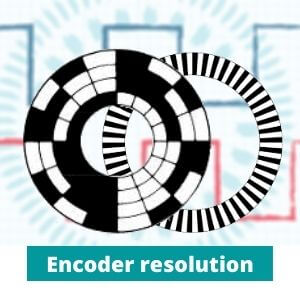## What is resolution of encoder?

Encoder resolution is a certain number of pulses that participate in a single working cycle. This parameter indicates the minimal shaft angle displacement the sensor can capture.

In rotary encoders, this value commonly determined by number of pulses per revolution, in linear ones by distance between two adjacent marks.

There are different types of encoders with various purposes. Let’s clarify the definition of resolution for each of the main categories.

## Incremental rotary encoder resolution

Absolute and incremental sensors have different purposes and therefore have different values of measurement. The differences in design we have already described here: Incremental vs absolute encoders. All types have similar principle and convert the mechanical motion into electrical signal.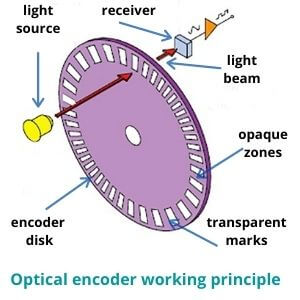Incremental sensors have identical slots and determine speed, acceleration and distance.

The most common signals used in encoders are sine-cosine (number of periods) and quadrature in the form of a square waves (number of pulses).

How to know the resolution of an encoder? Let's take a look how the optical quadrature encoder resolution is formed. The disk (code wheel) has two types of marks on its surface:

• Transparent (windows)
• Opaque (lines)

Such wheel rotates between the light source and the receiver. The light beam alternately hits windows or lines. When light passes through a transparent slot, the receiver records a high digital signal (1). When light enters an opaque slot, the receiver does not receive a pulse and records a low digital signal (0).

The incremental encoder angular resolution in this case shows the possible number of pulses (high signal) entering the receiver within one full disk revolution.

That is, if the receiver of the sensor can get 4192 pulses during one full disk rotation, the resolution of the quadrature encoder will be equal to 4192 counts per revolution.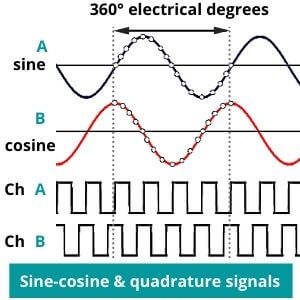The angular resolution of incremental encoder is specified in three most common values:

• (PPR) Pulses per revolution
• (LPR) Lines per revolution
• (CPR) Counts per revolution

Despite the various titles, their main purpose remains the same. Sinusoidal signals also have the same principle, but transmit not impulses, but periods. You can read more about how these values are formed here: Encoder PPR and CPR

The resolution of magnetic and optical encoders is defined in similar ways. Magnetic devices use magnetic poles as source, magnetic fields instead light beam and Hall sensor as a receiver.

High resolution incremental encoders are devices with more than 10000 ppr.

## Linear encoder resolution

The measuring principle of rotary and linear sensors is the same. But linear ones measure displacement along single line (axis). They use a scale with marks instead wheel with slots. A slider with built-in signal source and receiver moves under the scale.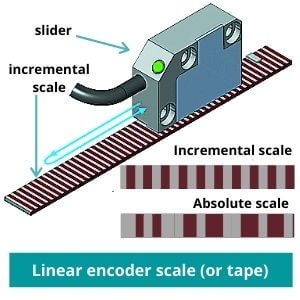Nevertheless, if the disk has a limitation of one cycle (360° mechanical degrees), the scale (strip) is limited only by its length. A special linear encoder tape can reach 100 m or even more. That is, we can't bind the measurement results to a single cycle.

Linear encoder resolution is determined by distance between two nearby marks (it is also called "steps"). The closer they are located, the more accurate measurement will be.

For example, sensor with step of 1 mm is much more accurate than sensor with step of 10 mm. The highest linear resolution can be measured even in microns (μm) or nanometers (nm). You may also encounter measures such as CPI (cycles per inch) or LPI (lines per inch).

## Absolute encoder resolution

How to find sensor resolution of absolute encoder? Absolute sensors have unique tracks for each position and measure particular location and displacement.

Rotary absolute encoders have disk that is dived into unique sectors. Each of this sectors keep coded information about its location in the wheel, using binary or grey code. Hence, absolute encoders send not pulses but coded information about current angular shaft position.

That is why the resolution of absolute sensors is measured not in pulses but in positons or codes per one full disk rotation. The more sectors the wheel will have the higher resolution would be.

However, the most often the resolution of absolute encoder is determined in bits. As you see, absolute encoders are all about data transmission. Amount of transmitted information is easier to calculate in bits.

Therefore, the resolution of absolute encoders is determined in bits per one cycle. If you need more information about data transmission, you will find it here: Serial vs parallel data transmission.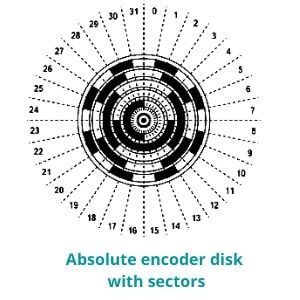Linear absolute encoders work at the similar principle. Coded position information is located on their scale and is measured in bits.

## Multiturn resolution

Sometimes you can see in specification two resolution values. In this case, you have a multiturn device.

There are two main types of absolute encoders:

• Single turn (ST)
• Multi turn (MT)

Single turn resolution is determined by number of bits during the revolution as described above.

Multiturn resolution is determined by number of bits, saving information about turns that have been made. In this case, the system collect data about amount of turns. Multiturn devices are always rotary.

## Encoder resolution calculation (formula)

How to calculate and choose encoder resolution? Before encoder resolution calculation, you need to know the minimum required offset. For example, you need to determine an offset of 6° degrees. So, encoder must give a high signal (pulse) every 6°. The disk has 360° mechanical degrees (in a single turnover). That is you need to use the following incremental encoder resolution formula: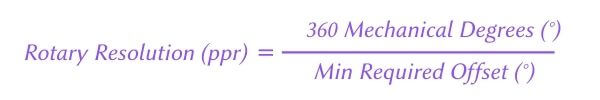360° /6° = 60 ppr.

If you already know how many pulses your encoder can receive during on cycle, you can calculate the smallest displacement that you can measure. Here is the formula: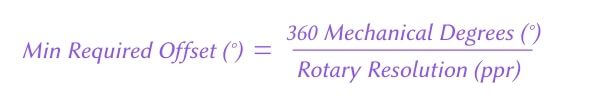If device have 4192 ppr it can measure displacement within 0.08° mechanical degree.

We have defined the lower possible limit. Now let`s calculate the maximum resolution. For this, you need to know the maximum rotation speed and frequency of the signal. The device will not work correctly above these parameters, so it is the upper allowed value.

To calculate the maximum resolution, use the formula: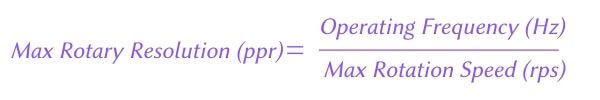For example, we have 3000 rotations per minute (rpm) and operating frequency 125 kHz. We need to translate this value to seconds (because Hz=s-1), so we have 50 rounds per second.

Now you need to divide the maximum frequency 125 kHz (125.000 Hz) by 50 rounds per second. In result, you have 2.500 ppr.

## Absolute encoder resolution calculation

The absolute encoder give feedback about the angular position by focusing on the code in its wheel sectors. To calculate the desired resolution you will also need to know the minimum required offset angle. Then you can calculate the minimum number of required sectors by the formula:For example, you need to measure displacement of 0.03 degrees. The disk have 360 mechanical degrees. Using the formula (360 / 0.03), you get 12.000 sectors (position per rotation or coders per rotation).

### How to convert bits into ppr?

Most often the resolution of absolute encoders is specified in bits. To quickly convert the number of bits into more understandable value (pulses or lines per revolution), you need to raise number 2 to the power of digit equal to the number of bits. For example, you have 12 bits that is equal 212 (4096 ppr). This formula is used with both single-turn and multi-turn resolution.

So, if you have 14 bits of singleturn and 16 bits of multiturn resolution that is equal 214 (16.384 ppr/cpr) and 216 (65.536 possible revolutions).

Here is the table with the most frequent values:

 Encoder Resolution (Bits) Encoder Resolution (ppr) 10 1.024 11 2.048 12 4.096 13 8.192 14 16.384 15 32.768 16 65.536 17 131.072 18 262.144 19 524.288 20 1.048.576

## Difference between Encoder Accuracy, precision and resolution

There are three main quality criteria for the encoder results:

• Resolution
• Accuracy
• Precision

These are different parameters and they are responsible for different aspects of measurement quality. We have already explained the first one, so let's take a closer look at the two remaining ones.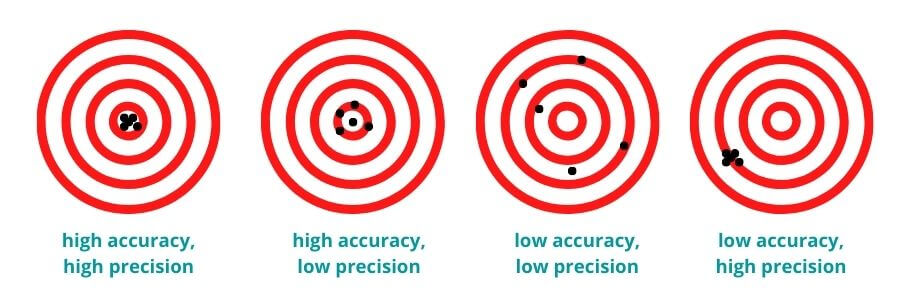### Accuracy vs resolution

Encoder accuracy is the difference between the actual measurement results and what the encoder shows to the controller.

A manufacturer indicates the maximum level of error tolerance in product specification. If the device have deviation from the true results more than specified in the characteristics, the sensor operates with an error.

Higher resolution will not give an advantage in simple operations. Sensors with 100 ppr and 100.000 ppr resolution will handle the task equally if you need to measure an offset within a degree.

However, if we are talking about microns and nanometers, a simpler sensor will not help anymore. A higher resolution sensor just makes it possible to work with less step.

Resolution parameter is always fixed (manufacturer already coded the disk). If your encoder resolution has changed without codding, then you have an error. However, external factors, incorrect mounting or wear may be affected to device accuracy.

### Encoder Precision

What is precision of encoder? For encoders, the term precision refers to the repeatability of results during repeated measurements.

All mechanisms wear out with time, it is an inevitable process. Repeatability is the ability to give the same accurate results after multiply cycles. This is important parameter for areas, where the constant accuracy of results is vital (conveyors or robotics).

Thank you for reading. We hope it helps. You can leave your feedback in comments section below.

Our company is official Eltra sensing technology distributor. On our website, you can easily select an Eltra encoder with the desired resolution by using filters. Check out our catalogue!

Name*
Email
2 + ? = 12